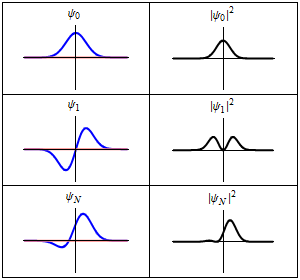# Mathematical physics facts for kids

Kids Encyclopedia FactsAn example of mathematical physics: solutions of Schrödinger's equation for quantum harmonic oscillators (left) with their amplitudes (right).

In applied mathematics, a branch of mathematics, mathematical physics refers to the knowledge made up of equations and ideas which scientists look to for assistance in modeling, describing, or solving problems in physics or related areas. These equations and ideas are derived from areas of pure mathematics. The equations form a consistent structure. An example of a so-called structure is a noncommutative space.

## Teachers of mathematical physics

There are many teachers that specialize in mathematical physics, one is Edward Witten, pictured here.

## Practitioners of mathematical physics

Practitioners includes those who develop and apply mathematical physics.

• von Neumann

## Associations of mathematical physics

There are several organized associations in mathematical physics, one is the International Association of Mathematical Physics.

## Images for kidsMathematical physics Facts for Kids. Kiddle Encyclopedia.